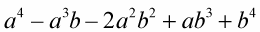##### Linear Algebra For DummiesDistributing a polynomial isn't hard. When distributing a polynomial over any number of other terms, you distribute each term in the first factor over all of the terms in the second factor. When the distribution is done, you combine anything that goes together to simplify.

The following example, (a + b + c + d + …)(z + y + x + w + …), is made up of only variables, none of which are the same.

1. Separate the terms in the first factor from one another, and multiply each term in the first factor times the second factor.

(a + b + c + d + …)(z + y + x + w + …) =

a(z + y + x + w + …) + b(z + y + x + w + …) + c(z + y + x + w + …) + …

2. Distribute and do the multiplication.

az + ay + ax + aw + … + bz + by + bx + bw + … + cz + cy + cx + cw + …

3. Combine like terms.

In this case, none of the terms are alike, but you should check.

The next example shows you how to multiply two trinomials.

1. Separate the terms in the first factor from one another; then multiply each term in the first factor times the second factor.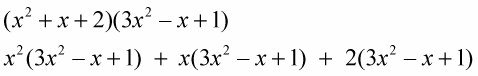2. Distribute and do the multiplication.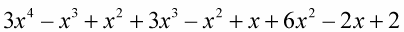3. Combine like terms.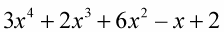Now trying solving an example where the first factor is a binomial and the second factor is a trinomial.

1. Separate the terms in the first factor from one another; then multiply each term in the first factor times the second factor.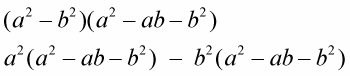2. Distribute and do the multiplication.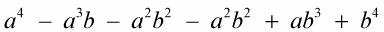3. Combine like terms.